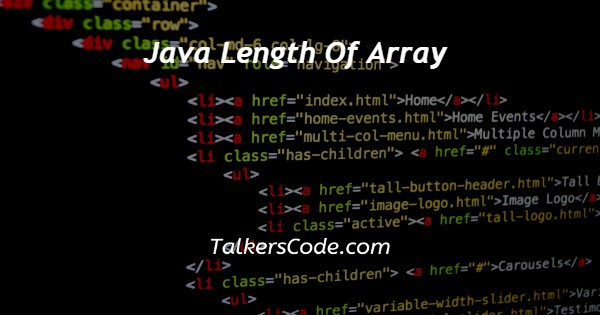# Java Length Of Array

Last Updated : Jul 1, 2023In this article we will show you the solution of java length of array, an array's length refers to the number of elements it can hold, and there are many types of lengths in Java. In order to obtain the length of an array, no predefined method exists.

Java gives us the ability to determine the length of an array using the array attribute length.

A name for the array is associated with this attribute. The purpose of this section is to demonstrate how to find the size or length of an array in Java.

An array's length can be determined by the attribute length provided by Java.

It is the size of an array that determines the length of the array inbuilt in every array.

Array size describes how many elements can be contained in a given array. By using the dot (.) operator followed by the array name, you can access the length property of an array.

We will now discuss the idea of how to define length of array java with an example.

## Step By Step Guide On Java Length Of Array :-

```public class TalkersCode
{
private static void LengthOfArray(String[] array)
{
//checks array is empty or not
if (array == null)
{
//if the array is empty prints the following statement
System.out.println("The array is empty, can't be determined length.");
}
else
{
//length attribute of the Array class determines the length of an array
int arrayLength = array.length;
//prints the array length
System.out.println("The length of the array is: "+arrayLength);
}
}
public static void main(String[] args)
{
String[] fruits = { "Paris", "Australia", "New york", "India", "USA"};
String[] alphabets = { "A", "I", "U", "l", "N" };
String[] numbers = { "15", "28", "53", "80", "60", "12", "44"};
//passing null value to the function
LengthOfArray(null);
//passing fruits array to the function
LengthOfArray(fruits);
//passing alphabets array to the function
LengthOfArray(alphabets);
//passing numbers array to the function
LengthOfArray(numbers);
}
}```
1. As part of our solution, we create a class called TalkersCode that has an array of strings as a parameter and a static method which takes in an array of strings.
2. In order to check if the array is empty or not, the LengthOfArray function is used. Arrays with empty values will print a statement stating that their length cannot be determined. An array's length is determined by its length attribute if it is not empty; otherwise, it is determined by its length attribute.
3. Three arrays of strings are created in the main method - fruit, alphabet, and number.
4. To test if the function can handle an empty array gracefully, we call the LengthOfArray function multiple times with different arrays as parameters.
5. Except for the null array, which prints out a statement saying it is empty, the program outputs the length of each array passed to the LengthOfArray method.

## Conclusion :-

As a result, we have successfully learned how to define length of array java with an example.

The Java Array Length attribute is very useful in various programming scenarios, which is why we have discussed it in this article.

In addition to discussing general cases, we discussed using arrays to find the largest and smallest value.

In addition, this attribute can be used for a variety of purposes. This length attribute can be useful in more cases, so we encourage you to find them and try it.

I hope this article on java length of array helps you and the steps and method mentioned above are easy to follow and implement.